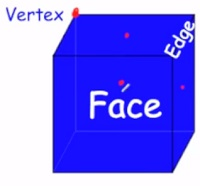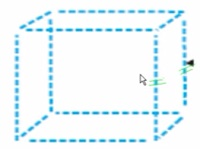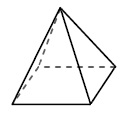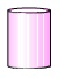# Vertices, Edges, and Faces of a Solid

Solid shapes have attributes like face, vertices, edges that describe them.Individual flat surfaces are called faces. Faces meet in straight lines called edges and points called vertices.

Edges − The line segments that form the skeleton of the 3D shapes are called edges.Faces − Flat surfaces enclosed by edges are called faces. A face of a solid shape is a plane or 2d figure.

Vertices − The corners where the edges meet are called vertices.

A rectangular prism has 6 faces 8 vertices and 12 edges.

A square pyramid has 5 faces 5 vertices and 8 edges.

Determine the faces, vertices and edges of the following shape.### Solution

Step 1:

The given shape is a square pyramid.

Step 2:

It has 5 faces, 5 vertices, and 8 edges.

Determine the faces, vertices and edges of the following shape.### Solution

Step 1:

The given shape is a cylinder.

Step 2:

It has 2 faces, 0 vertices, and 0 edges.